# Arrays in Java

## 1- What is Array?

In Java, array is a set of  elements with the same data type, and consecutive addresses on the memory. The array has the fixed number of elements and you can not change its size.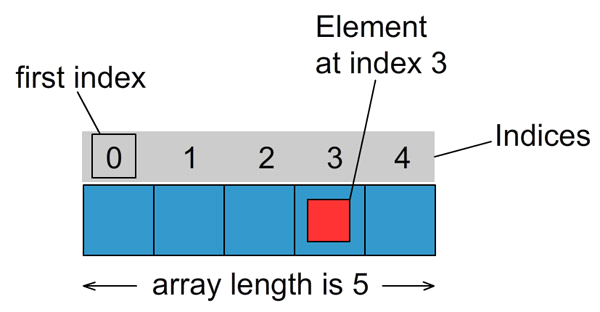The elements of an array are marked with a index, starting at index 0. You can access its elements through index.
Let's see, a array with 5 elements, int type.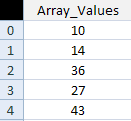In addition to one-dimensional arrays, you sometimes have to work with two-dimensional or multiple-dimensional arrays. For a two-dimensional array, its elements will be marked with two indexes such as row index and column index. Below is an image of a two- dimensional array.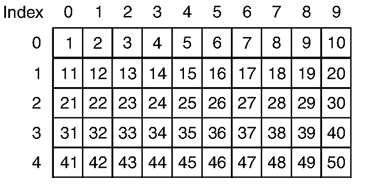## 2- One-dimensional array

How to declare an array in Java.
** Syntax **
``````
// Declare an array, not a specified number of elements.
int[] array1;

// Initialize the array with 100 elements
// The elements has not been assigned a specific value
array1 = new int;

// Declare an array specifies the number of elements
// The elements has not been assigned a specific value
double[] array2 = new double;

// Declare an array with elements assigned a specific values.
// This array has 4 elements
long[] array3= {10L, 23L, 30L, 11L};
``````
Let's look at an example: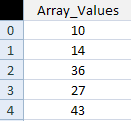ArrayExample1.java
``````
package org.o7planning.tutorial.javabasic.array;

public class ArrayExample1 {

public static void main(String[] args) {

// Declare an array with 5 elements
int[] myArray = new int;

// Note: the first element of the array index is 0:
// Assigning values to the first element (index 0)
myArray = 10;

// Assigning value to the second element (index 1)
myArray = 14;

myArray = 36;
myArray = 27;

// Assigning value to the 5th element (the last element in the array)
myArray = 18;

// Print out element count.
System.out.println("Array Length=" + myArray.length);

// Print to Console element at index 3 (4th element in the array)
System.out.println("myArray=" + myArray);

// Use a for loop to print out the elements in the array.
for (int index = 0; index < myArray.length; index++) {
System.out.println("Element " + index + " = " + myArray[index]);
}
}

}
``````
Result:
``````
Array Length=5
myArray=27
Element 0 = 10
Element 1 = 14
Element 2 = 36
Element 3 = 27
Element 4 = 18
``````
An illustrative example to use a for loop to assign values to the elements:
ArrayExample2.java
``````
package org.o7planning.tutorial.javabasic.array;

public class ArrayExample2 {

public static void main(String[] args) {

// Declare an array with 5 elements
int[] myArray = new int;

// Print out element count
System.out.println("Array Length=" + myArray.length);

// Using loop assign values to elements of the array.
for (int index = 0; index < myArray.length; index++) {
myArray[index] = 100 * index * index + 3;
}

// Print out the element at index 3
System.out.println("myArray = " + myArray);
}

}
``````
Result:
``````
Array Length=5
myArray = 903
``````

## 3- One-dimensional array and lặp for-each loop

Java 5 provides you with a for-each loop that allows you to traverse all elements of an array without using an index variable.
Example:
ArrayForeachExample.java
``````
package org.o7planning.tutorial.javabasic.array;

public class ArrayForeachExample {

public static void main(String[] args) {

// Declare an array of String.
String[] fruits = new String[] { "Apple", "Apricot", "Banana" };

// The for-each loop to traverse array.
for (String fruit : fruits) {

System.out.println(fruit);

}

}

}
``````
Running the example:
``````
Apple
Apricot
Banana
``````

## 4- The Utility methods for One-Dimensional Array

Note: You need to have knowledge of class and methods before seeing this section. Otherwise you can temporarily ignore it.
The Java provides you with some static methods for arrays, such as sorting arrays, assigning values to all array elements, searching, comparing arrays, etc. These methods are defined  in the Arrays class.
Below are some really useful, static methods defined in the Arrays class. These methods apply to the arrays of elements with byte, char, double, float, long, int, short, boolean types.
See more:
* Arrays *
``````
// Pass parameters of the same type, and returns a List.
public static <T> List<T> asList(T... a)

// Type X can be: byte, char, double, float, long, int, short, boolean
----------------------

// Search the index of a value that appears in the array.
// (Using binary search algorithm).
public static int binarySearch(X[] a, X key)

// Copy the elements of an array to create a new array with the specified length.
public static int[] copyOf(X[] original, X newLength)

// Copies the specified range of the specified array into a new array.
public static double[] copyOfRange(X[] original, int from, int to)

// Compare two arrays
public static boolean equals(X[] a, long[] a2)

// Assigns the same value to all elements of the array.
public static void fill(X[] a, X val)

// Convert an array to string.
public static String toString(X[] a)
``````
The following example uses some methods of the Arrays class.
ArraysExample.java
``````
package org.o7planning.tutorial.javabasic.array;

import java.util.Arrays;
import java.util.List;

public class ArraysExample {

public static void main(String[] args) {

int[] years = new int[] { 2001, 1994, 1995, 2000, 2017 };

// Sort
Arrays.sort(years);

for (int year : years) {
System.out.println("Year: " + year);
}

// Convert an array to string.

// Create lists from several values.
List<String> names = Arrays.asList("Tom", "Jerry", "Donald");

for (String name : names) {
System.out.println("Name: " + name);
}

}

}
``````
``````
Year: 1994
Year: 1995
Year: 2000
Year: 2001
Year: 2017
Years: [1994, 1995, 2000, 2001, 2017]
Name: Tom
Name: Jerry
Name: Donald
``````

## 5- Two-dimensional array

Very often, you have to work with an array, usually with a one-dimensional array. However, in case you want to work with tabular data, with a lot of columns and  rows, two-dimensional arrays should be used. For example, two-dimensional array will help you store information on chess-mans on a chessboard.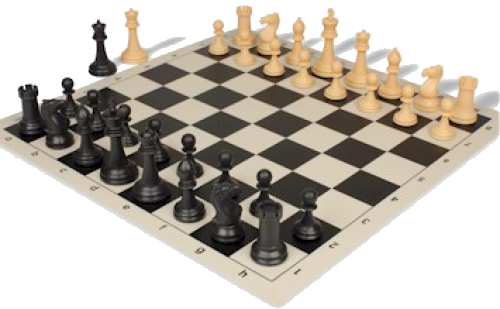The rows and columns of two-dimensional array are marked with indices 0, 1, 2,.... To access an element of a two- dimensional array, you need to access by two indices:  row index and column index.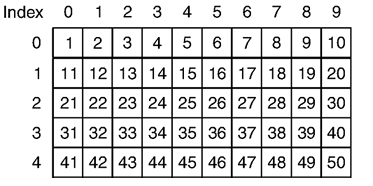length is a property of array. In case of two-dimensional array, this property is number of rows of the array.
You have three ways to declare a two-dimensional array.
``````
// Declare an array with 5 rows, 10 columns
MyType[][] myArray1 = new MyType;

// Declare 2 dimensional array with 5 row
// (Array of arrays)
MyType[][] myArray2 = new MyType[];

// Declare 2 dimensional array, Specifies the values for the elements.
MyType[][] myArray3 = new MyType[][] {

{ value00, value01, value02 , value03 },
{ value10, value11, value12 }
};

// ** Note:
// MyType can be either primitive (byte, char, double, float, long, int, short, boolean)
// or reference type.
``````
If you declare an array of primitive type (byte, char, double, float, long, int, short, boolean), the elements are not assigned values, but default values.
• Default value 0 corresponds to the types such as byte, double, float, long, int, short.
• Default value false corresponds to the boolean  type​​​​​​​.
• Default value '\u0000' (character null) corresponds to the char type.
Conversely, if you declare an array of reference type and if one element of the array is not assigned a value, it will be the null default value.
Example:
TwoDimensionalExample1.java
``````
package org.o7planning.tutorial.javabasic.array;

public class TwoDimensionalExample1 {

public static void main(String[] args) {

// Declare a two-dimensional array with 2 rows, 3 columns
// Elements have default values
int[][] myArray = new int;

// Row count of two dimensional array.
System.out.println("Length of myArray: "+ myArray.length); // ==> 2

// Print out
for (int row = 0; row < 2; row++) {

for (int col = 0; col < 3; col++) {
System.out.println("myArray[" + row + "," + col + "]=" + myArray[row][col]);
}
}
System.out.println(" --- ");

// Assigns values to elements.
for (int row = 0; row < 2; row++) {

for (int col = 0; col < 3; col++) {
myArray[row][col] = (row + 1) * (col + 1);
}
}

// Print out
for (int row = 0; row < 2; row++) {

for (int col = 0; col < 3; col++) {
System.out.println("myArray[" + row + "," + col + "]=" + myArray[row][col]);
}
}

}

}
``````
``````
Length of myArray: 2
myArray[0,0]=0
myArray[0,1]=0
myArray[0,2]=0
myArray[1,0]=0
myArray[1,1]=0
myArray[1,2]=0
---
myArray[0,0]=1
myArray[0,1]=2
myArray[0,2]=3
myArray[1,0]=2
myArray[1,1]=4
myArray[1,2]=6
``````
The two-dimensional array is actually an array of arrays.
In the Java, two-dimensional array is an array of arrays, therefore, you can declare a two- dimensional array simply by assigning the number of rows, without specifying the number of columns. Because the two-dimensional array is an "array of arrays", the length property of the two-dimensional array returns the number of rows in the array.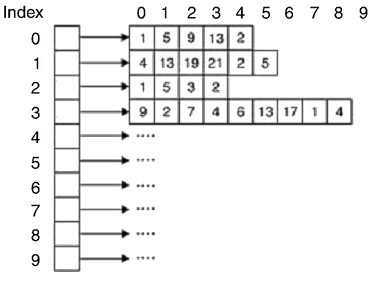TwoDimensionalExample2.java
``````
package org.o7planning.tutorial.javabasic.array;

public class TwoDimensionalExample2 {

public static void main(String[] args) {

// Declares a two-dimensional array, specifying the elements of the array.
String[][] teamAndPlayers = new String[][] {
{ "Sam", "Smith", "Robert" }, // US Players
{ "Tran", "Nguyen" }          // Vietnam Players
};

String[] usPlayers = teamAndPlayers;
String[] vnPlayers = teamAndPlayers;

System.out.println("Team count: " + teamAndPlayers.length); // ==> 2

System.out.println("Us Players count:" + usPlayers.length); // ==> 3
System.out.println("Vn Players count:" + vnPlayers.length); // ==> 2

for (int row = 0; row < teamAndPlayers.length; row++) {

String[] players = teamAndPlayers[row];

for (int col = 0; col < players.length; col++) {
System.out.println("Player at[" + row + "][" + col + "]=" + teamAndPlayers[row][col]);
}

}

}
}
``````
``````
Team count: 2
Us Players count:3
Vn Players count:2
Player at=Sam
Player at=Smith
Player at=Robert
Player at=Tran
Player at=Nguyen
``````
Example:
TwoDimensionalExample3.java
``````
package org.o7planning.tutorial.javabasic.array;

public class TwoDimensionalExample3 {

public static void main(String[] args) {

// Declare a Two-Dimensional Array (Array of arrays).
// Has 2 rows.
String[][] teamAndPlayers = new String[];

// US Players
teamAndPlayers = new String[]{ "Sam", "Smith", "Robert" };

// Vietnam Players
teamAndPlayers = new String[]{ "Tran", "Nguyen" };

for (int row = 0; row < teamAndPlayers.length; row++) {

String[] players = teamAndPlayers[row];

for (int col = 0; col < players.length; col++) {
System.out.println("Player at[" + row + "][" + col + "]=" + teamAndPlayers[row][col]);
}

}

}
}
``````
``````
Player at=Sam
Player at=Smith
Player at=Robert
Player at=Tran
Player at=Nguyen
``````

#### View more Tutorials:

Maybe you are interested

These are online courses outside the o7planning website that we introduced, which may include free or discounted courses.

•Learn and Understand Interfaces in C#
•Learn JavaScript From Scratch:Become Top Rated Web Developer
•Java Object-Oriented Programming : Build a Quiz Application
•Struts 2 Framework for Beginners
•HTML 5 and CSS 3 - tricks and workarounds
•Learn AngularJS Step By Step
•Learning Path: Bootstrap: The Complete Guide to Bootstrap 4
•HTML5 + CSS3 + Bootstrap: The Beginner Web Design Course
•*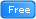* Java Database Connection: JDBC and MySQL
•iOS 13 - How to Make Amazing iPhone Apps: Xcode 11 & Swift 5
•Advanced Java programming with JavaFx: Write an email client
•Create Android and iOS App using HTML, CSS and JS
•Spring Boot Microservices with JPA
•React, Redux, & Enzyme - Introducing Apps & Tests
•Learn C++ Game Development
•Java JDBC with Oracle: Build a CRUD Application
•** MongoDB Essentials - Understand the Basics of MongoDB
•RESTful Web API Design with NodeJS
•MySQL database, MySQLi class, Essentials and Much Much More
•JavaScript and React for Developers: Master the Essentials
•SDLC with SOA Spring Java JMS GIT ActiveMQ MongoDB Jenkins
•Complete Step By Step Java For Testers
•Understanding JDBC with PostgreSQL (A step by step guide)
•** Build and Deploy a LAMP server on AWS